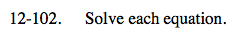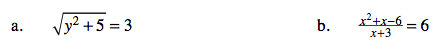### Home > AC > Chapter 12 > Lesson 12.4.4 > Problem12-102

12-102.Square both sides of the equation.

Solve for y.

Multiply both sides of the equation by (x + 3).

Set the equation equal to zero.

Solve for x.